# Sinusoidal Graph Drawing Problem

#### cikalekli

Joined Dec 10, 2020
103
The was an equation given like that:
v(t) = 5cos(1256t-pi/4)

Then I wanted to draw this graph below: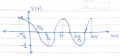Do I need to scroll the chart left or right? (In the cosine graph, I shifted it to the left side)

Also I want to ask that, I have been always encountered with sine function graph drawings.
So, should I always first convert my equation to the sine function to draw the graph?
Or, may I use the idea below: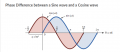#### MrChips

Joined Oct 2, 2009
28,127
In order to help you draw the waveform, try to find a few points to get started.

1) Find the value of v(0). This will give you your first point (v, 0).

2) Find the first value of t for v(t) = 0, for another point (0, t).

3) Find the value of (vmax, t) for the first occurrence.

4) Find the value of (-vmax, t) for the first occurrence.

5) Find the second value of t for v(t) = 0.

Put these points on your graph paper. Now you ought to be able to draw the waveform.

•cikalekli

#### cikalekli

Joined Dec 10, 2020
103
In order to help you draw the waveform, try to find a few points to get started.

1) Find the value of v(0). This will give you your first point (v, 0).

2) Find the first value of t for v(t) = 0, for another point (0, t).

3) Find the value of (vmax, t) for the first occurrence.

4) Find the value of (-vmax, t) for the first occurrence.

5) Find the second value of t for v(t) = 0.

Put these points on your graph paper. Now you ought to be able to draw the waveform.
Thank you, according to the substances you said my drawing hasn't change.

However, I am really wondering that as far as I know that -pi/4 means the graph supposed to be shifted to the right...

Why my graph resulted with the shift of to the right?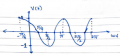#### Papabravo

Joined Feb 24, 2006
19,845
A negative phase angle will shift the graph to the left, as your drawing has indicated.
A positive phase angle will shift the graph to the right. There is a limit. If the phase angle is 2π, then the graph will appear "unshifted" which is the essence of the definition of a periodic function.

•cikalekli

#### cikalekli

Joined Dec 10, 2020
103
A negative phase angle will shift the graph to the left, as your drawing has indicated.
A positive phase angle will shift the graph to the right. There is a limit. If the phase angle is 2π, then the graph will appear "unshifted" which is the essence of the definition of a periodic function.
Ah thanks a lot... Now I got it but in my book (Electric Circuits - James Nilsson) is told different about it: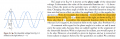Why it says if phase angle is negative -------> shift right
If it is positive ------->shift left

EDIT:
Here I tested the equation from desmos.com by only changing angular freq. without touching the value of phase angle:Here the program shifted it to the right...

I got confused so much and I just wanna learn the right one.

#### MrChips

Joined Oct 2, 2009
28,127
Let's look at a sine function.
When there is no phase shift (Φ = 0), the wave crosses at the origin (0, 0).
If you add a positive phase shift, you are moving up in voltage v(t) at t = 0.

With positive phase shift, you are moving the vertical axis (t = 0) to the right on the waveform.
Relative to the graph it appears as if the waveform is moving to the left.
Hence positive phase is shifting to the LEFT on the graph.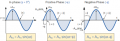•cikalekli

#### cikalekli

Joined Dec 10, 2020
103
Let's look at a sine function.
When there is no phase shift (Φ = 0), the wave crosses at the origin (0, 0).
If you add a positive phase shift, you are moving up in voltage v(t) at t = 0.
Hence positive phase is shifting to the LEFT.
Ah alright, the last thing is you in the equation the pi/4 was given with a negative form.
If it was given like +pi/4, then is it gonn be shifted to the right then?

I mean yeah I got you about the thing of "positive phase is shifting to the left"

However, when they give us the phase angle in the equation, don't we pay attention to the positivity or negativity at the beginning of the phase angle?

thanks again

#### MrChips

Joined Oct 2, 2009
28,127
I have edited my post.

When you add a positive phase shift you are moving the vertical axis (t = 0) to the right.

•MrAl

#### RBR1317

Joined Nov 13, 2010
706
Then I wanted to draw this graph below:
If you draw the graph with the X-axis measured in radians rather than seconds, then what was the purpose of specifying the frequency?

•cikalekli

#### cikalekli

Joined Dec 10, 2020
103
If you draw the graph with the X-axis measured in radians rather than seconds, then what was the purpose of specifying the frequency?
Yeah you right but I just stuck at the shifting the equations of sin and cos waves#### cikalekli

Joined Dec 10, 2020
103
Let's look at a sine function.
When there is no phase shift (Φ = 0), the wave crosses at the origin (0, 0).
If you add a positive phase shift, you are moving up in voltage v(t) at t = 0.

With positive phase shift, you are moving the vertical axis (t = 0) to the right on the waveform.
Relative to the graph it appears as if the waveform is moving to the left.
Hence positive phase is shifting to the LEFT on the graph.
Regarding of this image:
View attachment 239574

When the phase is positive, they shifted it to the left...

Is this rule is different for cosine and sine functions?

Because my equation was cosine and once the phase angle is given negative, we again shifted to the left...

#### ericgibbs

Joined Jan 29, 2010
17,147
hi cika,
The -pi/4.
Consider if the above was not part of the equation
v(t) = 5cos(1256t)
If you plotted that, the plot would start as a Vmax at time=0
So consider a time earlier than time zero on the Cosine plot, when you sub the pi/4

Perhaps this sim will give you a clue.
E

#### cikalekli

Joined Dec 10, 2020
103
hi cika,
The -pi/4.
Consider if the above was not part of the equation
v(t) = 5cos(1256t)
If you plotted that, the plot would start as a Vmax at time=0
So consider a time earlier than time zero on the Cosine plot, when you sub the pi/4

Perhaps this sim will give you a clue.
E
Oh with LTspice view, that looked crystal clear understanding.

So, to wrap it up if the equation was given like,

5cos(1256t-pi/4) or 5sin(1256t-pi/4)

In both of the cos or sin situations, we shift to the right.

Did I conclude it correctly please?

#### ericgibbs

Joined Jan 29, 2010
17,147
hi C,
Don't over think the problem.
At time =0 the numeric value of time of either sin(t-pi/4) or cos(t-pi/4) must earlier than time zero.
I cannot give you the answer.
E

#### Attachments

•cikalekli

#### cikalekli

Joined Dec 10, 2020
103
hi C,
Don't over think the problem.
At time =0 the numeric value of time of either sin(t-pi/4) or cos(t-pi/4) must earlier than time zero.
I cannot give you the answer.
E
Ah again Eric you saved my life sir. Now understood it clearly with those simulation demonstrations... ^_^

#### RBR1317

Joined Nov 13, 2010
706
In both of the cos or sin situations, we shift to the right.
It seems that I can never remember those kind of rules, but I can find the zero point:

(1256t-π/4)=0

Solve for "t" and that is the point where sin()=0 & cos()=1.

•cikalekli

#### MrChips

Joined Oct 2, 2009
28,127
Ah again Eric you saved my life sir. Now understood it clearly with those simulation demonstrations... ^_^
As Eric says, don't overthink the problem.
It does not matter if it is a sine wave, cosine wave or any random waveform.

It is relative. It depends on if your perspective is with respect to the waveform or with respect to the graph.
Forget about trying to learn which rule applies because you will not remember which rule to use.

Instead, think of time t = 0.
You are moving the goalpost, t = 0.
If you add a positive number to t, then you are moving the goal post to a positive time (time into the future).

•cikalekli

#### cikalekli

Joined Dec 10, 2020
103
As Eric says, don't overthink the problem.
It does not matter if it is a sine wave, cosine wave or any random waveform.

It is relative. It depends on if your perspective is with respect to the waveform or with respect to the graph.
Forget about trying to learn which rule applies because you will not remember which rule to use.

Instead, think of time t = 0.
You are moving the goalpost, t = 0.
If you add a positive number to t, then you are moving the goal post to a positive time (time into the future).
You are really right and I'm not gonna overthink again for that. I also liked the analogy you made. Catchy and useful.

#### MrAl

Joined Jun 17, 2014
9,773
Hello there,

Here is another quick view of how the phase shift affects the wave shift in time.

If we have a sine wave like:
y=A*sin(w*t+ph)

we see inside the parentheses that w*t and ph are being added, and that must mean that they have the same dimensions. Since "w*t" is in units of frequency times the time, "ph" (the phase shift) must also be in units of time, but because the phase shift "ph" is constant and "w" is constant, the time for that part must also be constant. So we can equate the following:
ph=T*w

where T is a constant time value.
Now to calculate T, all we have to do is solve for T in that little equation, and we get:
T=ph/w

The final form then is:
A*sin(w*t+w*T)

and factoring inside we get:
A*sin(w*(t+T))

and now we can immediately see that any positive phase shift "adds" to the time value and thus makes the wave appear as if it is starting at some positive time value even when lower case "t" is zero.

I like the idea about "positvie phase shift moves the 'y' axis in the positive direction:" though read earlier in this thread, i'll have to give that post a likeThere is one little catch however with any of these views, and that is when the phase shift is greater than 360 degrees we may see the opposite happen, depending on the actual value of the phase shift. That is because for many applications especially in filtering we often truncate the phase shift to angles less than 360 degrees. So if the original phase shift is positive and greater than 360 degrees we might see the y axis actually shift to the left. That's only a concern for angles over 360 degrees, and it wont always happen it depends on the actual value of the larger phase shift.
Do a couple and graph them and see for yourself, it's interesting.
Also keep in mind that for some applications even though we may see the plot shift the opposite way in an angle truncated expression we might still have to call it positive (or negative) because that kind of application may require that we maintain the entire phase shift rather than truncate it to an angle less than 360 degrees. This is a bit rare but still possible.